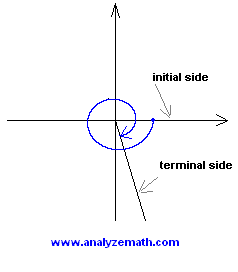Trigonometry questions related to angles in standard position, coterminal angles, complementary and supplementary angles, as well conversion from degrees to radians and vice versa, are presented. The solutions and answers are provided.

Question 1

Graph - 435o in standard position.

Solution to Question 1:

• Start from the initial side on the horizontal axis, positive direction, rotate 435 degrees in the negative direction to locate the terminal side which is in quadrant four. It helps to note that 435 degrees = 360 degrees + 75 degreesQuestion 2

Graph 9 Pi / 4 in standard position.

Solution to Question 2:

• Start from the initial side on the horizontal axis, positive direction, rotate 9 Pi / 4 (radians) in the positive direction to locate the terminal side which is in quadrant one. Note that 9 Pi / 4 = 2 Pi + Pi / 4.Question 3

In which quadrant is the terminal side of an angle of - 3 Pi / 4 located?

Solution to Question 3:

• The terminal side of - 3 Pi / 4 is located in quadrant three.

Question 4

In which quadrant is the terminal side of an angle of 750o located?

Solution to Question 4:

• 750 degrees = 360 degrees + 360 degrees + 30 degrees. Hence an angle of 750 degrees, in standard position, has its terminal side in quadrant one.

Question 5

Find a coterminal angle t to angle - 27 Pi / 12 such that 0 <= t < 2 Pi.

Solution to Question 5:

• We first note that - 27 Pi / 12 = -24 Pi / 12 - 3 Pi / 4 = - 2 Pi - 3 Pi / 4. A coterminal angle is obtained by adding or subtracting a whole number of 2 Pi (or 360 degrees). Hence a positive coterminal angle to - 27 Pi / 12 may be obtained by adding 2 (2 Pi) = 4 Pi.

t = - 27 Pi / 12 + 4 Pi = 7 Pi / 4

• Note that t is positive and smaller than 2 Pi..

Question 6

Find an angle t that is coterminal to 560o such that 0 <= t < 360o.

Solution to Question 6:

• Note that 560 degrees = 360 degrees + 200 degrees which is greater than 360 degrees. So to obtain a coterminal angle smaller than 360 degrees we need to subtract 360 degrees from 560 degrees.

t = 560 degrees - 360 degrees = 200 degrees.

Question 7

Determine the complementary angle t to Pi / 12.

Solution to Question 7:

• The complementary angle to Pi / 12 is obtained as follows

t = Pi / 2 - Pi / 12 = 5 Pi / 12

Question 8

Determine the complementary angle t to 34o.

Solution to Question 8:

• The complementary angle t to 34 degrees is given by

t = 90 degrees - 34 degrees = 56 degrees.

Question 9

Determine the supplementary angle t to 96o.

Solution to Question 9:

• The supplementary angle t to 96 degrees is give by

t = 180 degrees - 96 degrees = 84 degrees.

Question 10

Solution to Question 10:

• To convert from degrees to radians, we multiply by Pi and divide by 180. Hence 75 degrees in radians is given by

75 * Pi / 180 = 5 Pi / 12 = 1.31 (rounded to 2 decimal places)

Question 11

Convert 7 Pi / 4 to degrees .

Solution to Question 11:

• To convert from radians to degrees, we multiply by 180 and divide by Pi. Hence 7 Pi / 4 in degrees is given by

(7 Pi / 4) * 180 / Pi

• which simplifies to

= 315 degrees.

Question 12

Solution to Question 12:

• 1.5 radians into degrees is given by

1.5 * 180 / Pi = 85.94 degrees (rounded to 2 decimal places)

Question 13

Convert 61o 05' 12" to degrees in decimal form.

Solution to Question 13:

• An angle of 61 degrees 5 minutes and 12 seconds in decimal form is given by

61 + 5 / 60 + 12 / 3600 = 61.07 (rounded to 2 decimal places).

Question 14

A central angle t of a circle with radius 2 meters subtends an arc of length 1.5 meters. Find angle t in degrees.

Solution to Question 14:

• The use of the arc length formula s = r t where t is the angle (in radians) that subtends an arc of length s gives

1.5 = 2 t

• Solve for t to obtain

t = 1.5 / 2 = 0.75 (radians)

• We now convert t in degrees

t = 0.75 * 180 / Pi = 42.97 degrees (rounded to 2 decimal places)